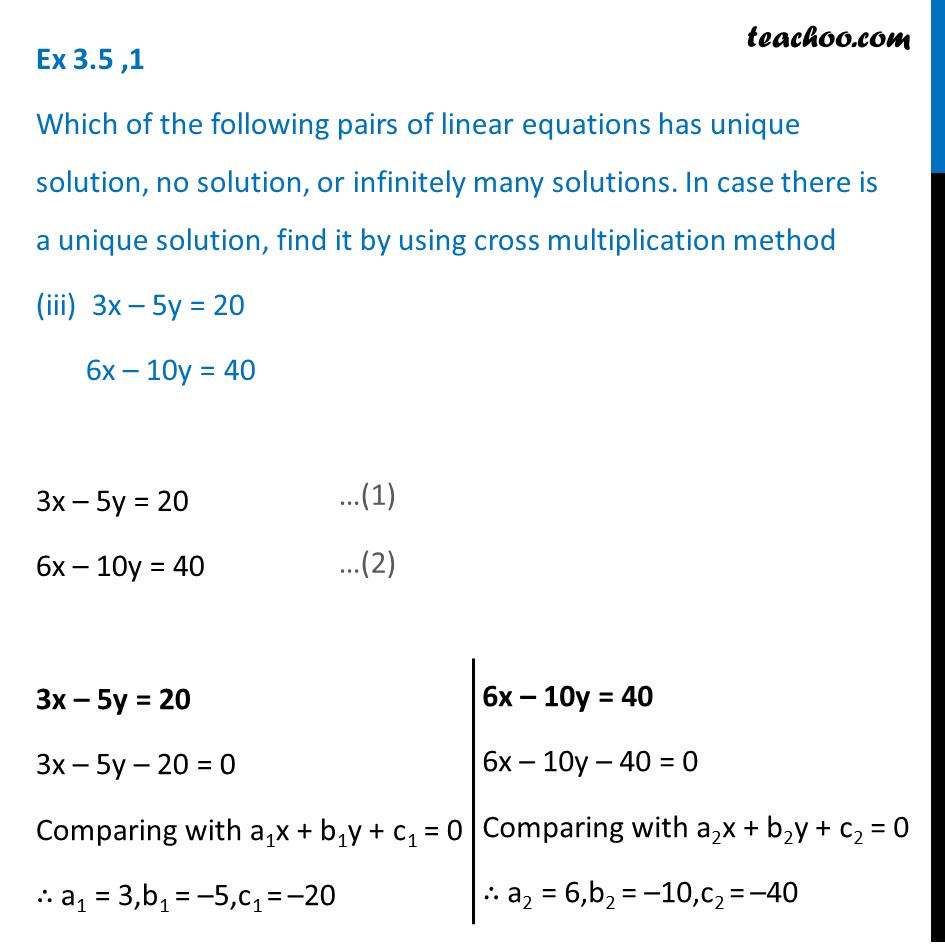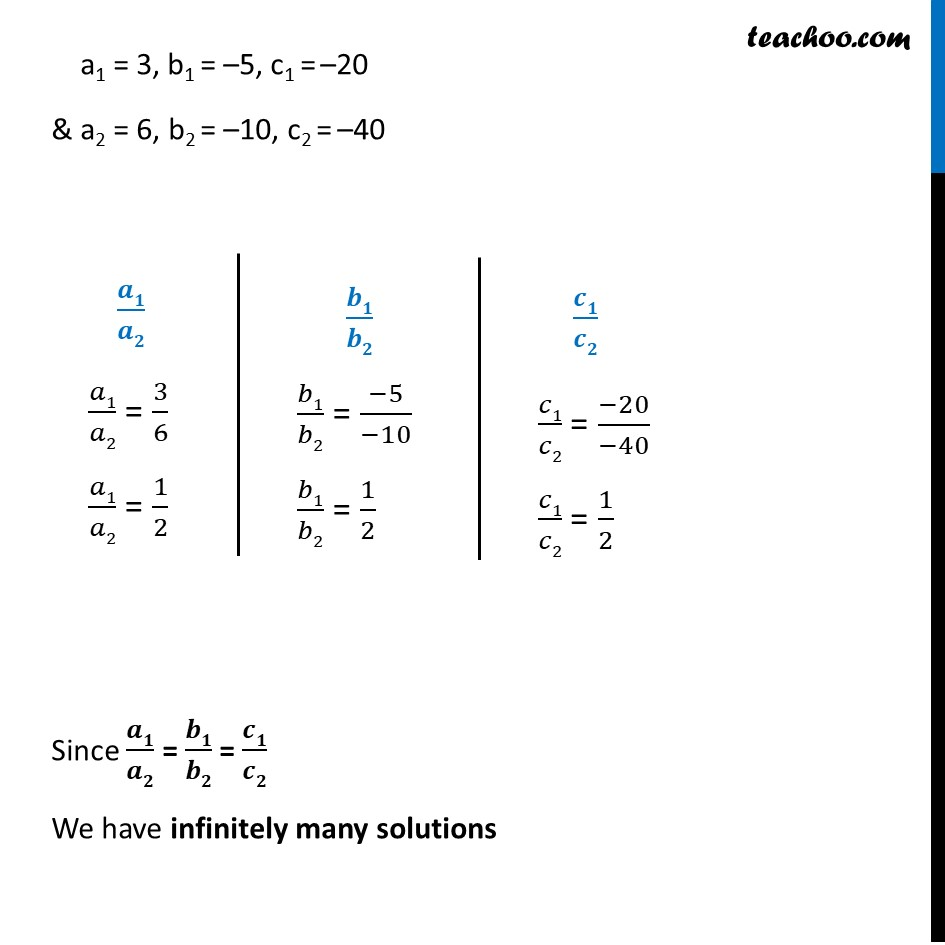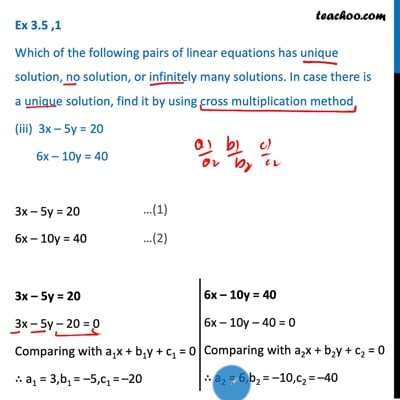Ex 3.5

Chapter 3 Class 10 Pair of Linear Equations in Two Variables
Serial order wiseThis video is only available for Teachoo black users

Introducing your new favourite teacher - Teachoo Black, at only ₹83 per month

### Transcript

Ex 3.5 ,1 Which of the following pairs of linear equations has unique solution, no solution, or infinitely many solutions. In case there is a unique solution, find it by using cross multiplication method (iii) 3x – 5y = 20 6x – 10y = 40 3x – 5y = 20 6x – 10y = 40 3x – 5y = 20 3x – 5y – 20 = 0 Comparing with a1x + b1y + c1 = 0 ∴ a1 = 3,b1 = –5,c1 = –20 6x – 10y = 40 6x – 10y – 40 = 0 Comparing with a2x + b2y + c2 = 0 ∴ a2 = 6,b2 = –10,c2 = –40 a1 = 3, b1 = –5, c1 = –20 & a2 = 6, b2 = –10, c2 = –40 𝒂𝟏/𝒂𝟐 𝑎1/𝑎2 = 3/6 𝑎1/𝑎2 = 1/2 𝒃𝟏/𝒃𝟐 𝑏1/𝑏2 = (−5)/(−10) 𝑏1/𝑏2 = 1/2 𝒄𝟏/𝒄𝟐 𝑐1/𝑐2 = (−20)/(−40) 𝑐1/𝑐2 = 1/2 Since 𝒂𝟏/𝒂𝟐 = 𝒃𝟏/𝒃𝟐 = 𝒄𝟏/𝒄𝟐 We have infinitely many solutions